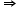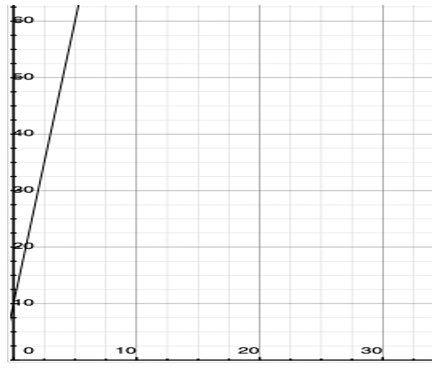Maths-
General
Easy

Question

# A baker has already made 10 cakes . She can make the same number of cakes each hour , which she does for 5 hours.  Sketch the graph of the relationship between the number of cakes made and timeHint:

## The correct answer is: end point of the graph is (5 , 60).

### SOL – Let no of cakes made = yTime taken = y hoursAcc. to the question, a baker has already made 10 cakesWhen time, x = 0, no of cakes, y = 10y – intercept, c = 10We know that slope is given by rate of change.And it is given that she can make 10 cakes per hour.Slope of the line = 10Using slope – intercept form, equation of line is y = mx + cy = 10x + 10Also, she made cake for 5 hours i.e., x = 5y = 10(5) + 10= 50 + 10 = 60So, end point of the graph is (5 , 60).#### With Turito Foundation.#### Get an Expert Advice From Turito.